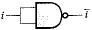# Boolean Algebra And Logic Gates (Advance Level)- 1

## 15 Questions MCQ Test Question Bank for GATE Computer Science Engineering | Boolean Algebra And Logic Gates (Advance Level)- 1

Description
This mock test of Boolean Algebra And Logic Gates (Advance Level)- 1 for Computer Science Engineering (CSE) helps you for every Computer Science Engineering (CSE) entrance exam. This contains 15 Multiple Choice Questions for Computer Science Engineering (CSE) Boolean Algebra And Logic Gates (Advance Level)- 1 (mcq) to study with solutions a complete question bank. The solved questions answers in this Boolean Algebra And Logic Gates (Advance Level)- 1 quiz give you a good mix of easy questions and tough questions. Computer Science Engineering (CSE) students definitely take this Boolean Algebra And Logic Gates (Advance Level)- 1 exercise for a better result in the exam. You can find other Boolean Algebra And Logic Gates (Advance Level)- 1 extra questions, long questions & short questions for Computer Science Engineering (CSE) on EduRev as well by searching above.
QUESTION: 1

### The function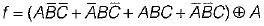can be written as

Solution: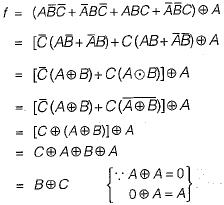QUESTION: 2

### The Karnaugh map for the Boolean function F of 4 Boolean variables is given below where A, B, C are don't care conditions. What values of A, B, C will result in the minimal expression?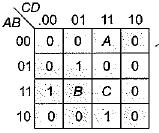Solution:

From the given k-map we can observe that B = 1 always. Also if A is to be paired than it should be
1. If A is selected there is no need to take C.
Hence, A - 1, B = 1, C = 0 Hence option (d) is correct

QUESTION: 3

### Let f(A, B) =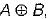then simplified from of the function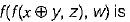Solution: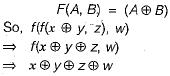QUESTION: 4

Consider the circuit-shown below. Each of the control inputs, C0 through C3, must be tied to a constant, either ‘0’ or '1'.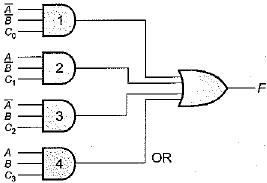What are the values of C0 through C3 that would cause F to be the exclusive OR of A and B?

Solution:

The output of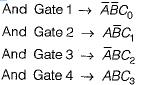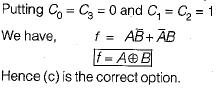QUESTION: 5

Write the minimized expression of the function of three variables that is 1 if the third variable is equal to the OR of the first two variable, 0 otherwise

Solution:

Constructing the table we have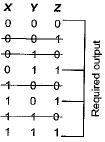Hence the required expression is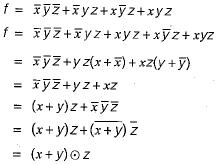QUESTION: 6

Which of the following functions implements the Karnaugh map shown below?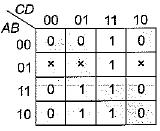Solution:

Solving the given K-map we have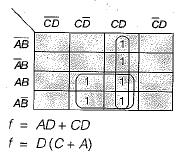Hence (b) is correct option

QUESTION: 7

The Boolean expression for the shaded area in the given Venn diagram is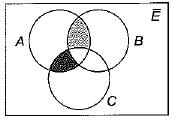Solution:

Hence, the required boolean expression is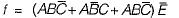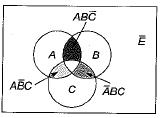Hence (b) is the required option.

QUESTION: 8

The Boolean expression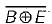is a simplified version of expression: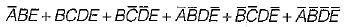Then which of the following choice is correct:
1. Don’t care conditions don’t exist.
2. Don’t care conditions exist.
3. D (16, 18, 20, 23, 27, 29) is the set of don’t care conditions.
​4. D (16, 20, 22, 27, 29) is the set of don’t care conditions.

Solution: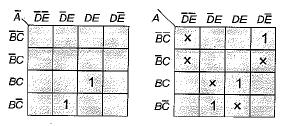Only (c) option satisfies the required condition.

QUESTION: 9

The logical expression for K-Map shown above is: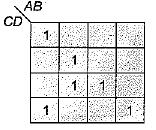Solution: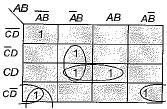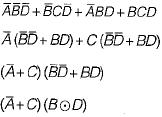QUESTION: 10

The Boolean expression for the shaded area in the Venn diagram is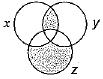Solution: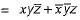QUESTION: 11

Consider, a four variable Boolean function, which contains half the number of minterms with odd number of 1 ’s. Then the Boolean can be realized with variables A, B, C, D as:

Solution:

Constructing the K-map we have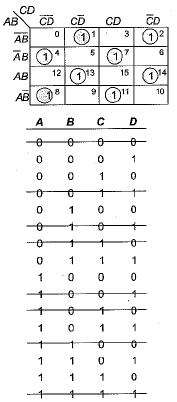Hence the required output is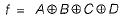QUESTION: 12

Consider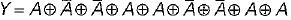the Y is equivalent to:

Solution: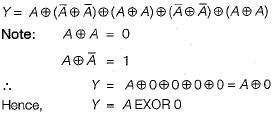QUESTION: 13

An X-Y flip-flop, whose characteristic table is given below, is to be implemented using a JK flip-flop. This can be done by making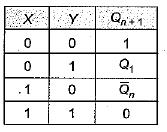Solution: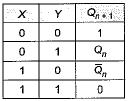The JK fiip-flop can be implemented by making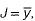, K = x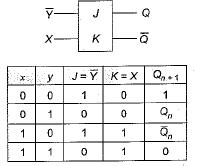Which is true
Hence (d) is correct option.

QUESTION: 14

For what logic gate, the output is complement of the input?

Solution:

By using NOT gate output is complement of input

QUESTION: 15

The NAND can function as a NOT gate if

Solution:

NAND gate work as NOT if inputs are connected together i.e.